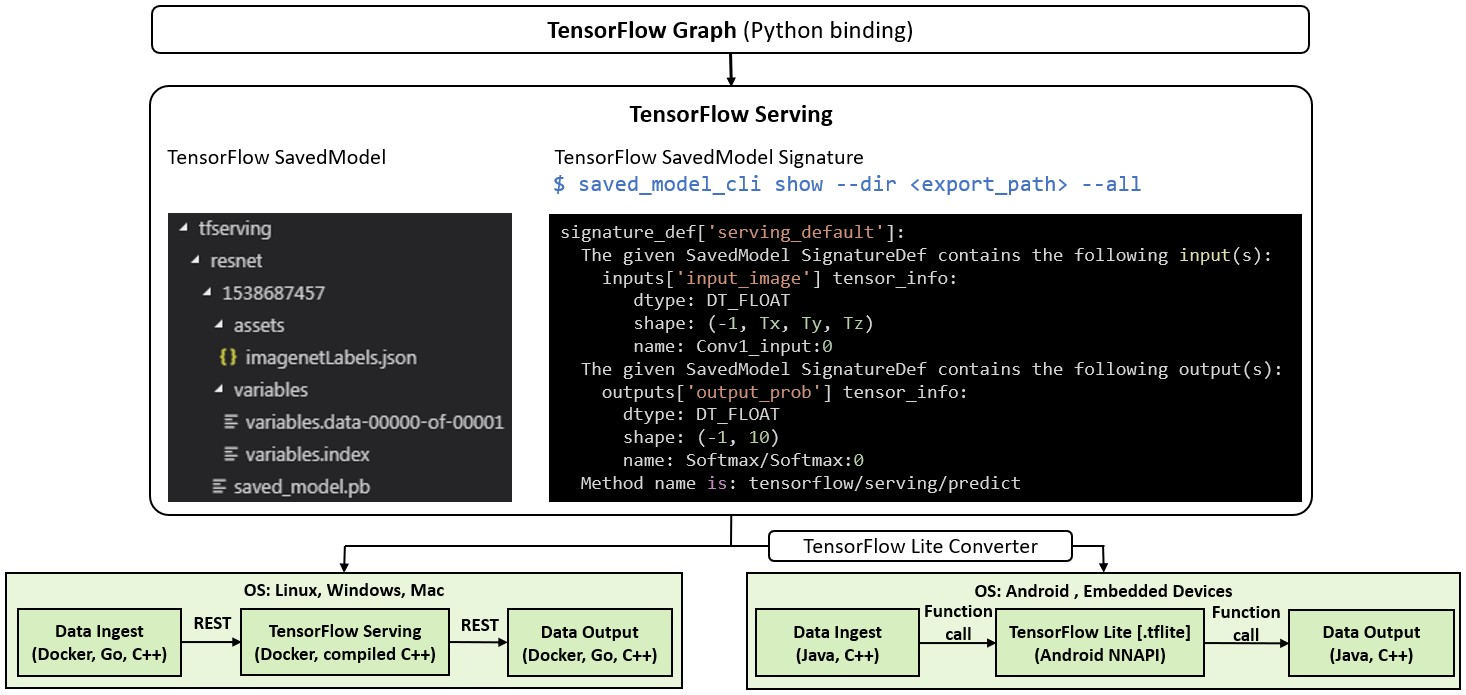# TensorFlow

Every machine learning project must produce a saved model as its final deliverable.

## Keras

• Do not import libraries directly from the Keras package such as
  import keras.utils


Instead, always import from the Tensorflow package. For example,

  import tensorflow.keras.utils


## SavedModel

• Contains complete architecture and weights.
• Independent of the source code that created the model.
• Suitable for deployment via TensorFlow Serving, TensorFlow Lite, in many programming languages (e.g., C++, Java, Go)
• A saved model produces a folder consisting of the following files:
  tfserving                                           # TensorFlow Serving
├── cnn                                             # model name
|    ├── 1                                          # model version
|    |   ├── assets/
|    |   ├── variables/
|    |   |   ├── variables.data-*****-of-*****
|    |   |   └── variables.index
|    |   └── saved_model.pb
|    └── 2                                          # model version
|        ├── assets/
|        ├── variables/
|        |   ├── variables.data-*****-of-*****
|        |   └── variables.index
|        └── saved_model.pb
└── mtcnn                                           # model name
└── 1                                           # model version
├── assets/
├── variables/
|   ├── variables.data-*****-of-*****
|   └── variables.index
└── saved_model.pb

• The .pb is the MetaGraphDef which holds the graph structure. MetaGraph is a dataflow graph, plus its associated variables, assets, and signatures. A MetaGraphDef is the protocol buffer representation of a MetaGraph.
• The variables folder holds your learned weights.
• The assets folder allow you to add external files that may be needed.
• Refer to the official tutorial for saving and loading a TensorFlow model.
• A simplified example of writing TensorFlow machine learning model and saving it into SavedModel in Python is given below.
  import tensorflow as tf
from keras.models import Model
from keras.layers import Input, Conv2D, Activation, Flatten, Dense,

. . .

# TensorFlow graph
x_input = Input(shape=(Tx, Ty, Tz)) # Desired shape of input data
x_act = Activation('relu')(x_conv)
x_flat = Flatten()(x_act)
x_output = Dense(10, activation='softmax')(x_flat)
model = Model(inputs=x_input, outputs=x_output, name='Classifier')

# Define an optimizer, compile, and fit the model

  \$ saved_model_cli show --dir /<path to tfserving saved model>/tfserving/resnet/1538687457 --all